Math Olympiad Test: Statistics- 2

# Math Olympiad Test: Statistics- 2

Test Description

## 15 Questions MCQ Test Mathematics Olympiad for Class 9 | Math Olympiad Test: Statistics- 2

Math Olympiad Test: Statistics- 2 for Class 9 2022 is part of Mathematics Olympiad for Class 9 preparation. The Math Olympiad Test: Statistics- 2 questions and answers have been prepared according to the Class 9 exam syllabus.The Math Olympiad Test: Statistics- 2 MCQs are made for Class 9 2022 Exam. Find important definitions, questions, notes, meanings, examples, exercises, MCQs and online tests for Math Olympiad Test: Statistics- 2 below.
Solutions of Math Olympiad Test: Statistics- 2 questions in English are available as part of our Mathematics Olympiad for Class 9 for Class 9 & Math Olympiad Test: Statistics- 2 solutions in Hindi for Mathematics Olympiad for Class 9 course. Download more important topics, notes, lectures and mock test series for Class 9 Exam by signing up for free. Attempt Math Olympiad Test: Statistics- 2 | 15 questions in 15 minutes | Mock test for Class 9 preparation | Free important questions MCQ to study Mathematics Olympiad for Class 9 for Class 9 Exam | Download free PDF with solutions
 1 Crore+ students have signed up on EduRev. Have you?
Math Olympiad Test: Statistics- 2 - Question 1

### The no. of class intervals, if the magnitude of class interval is 4 will be: Data: 31, 23, 19, 29, 20, 16, 22, 10, 13, 34, 33, 38, 36, 24, 18, 15, 12, 30, 27, 23, 20

Detailed Solution for Math Olympiad Test: Statistics- 2 - Question 1

Range = 38 – 10 = 28
∴ number of class intervals = 28/4 = 7

Math Olympiad Test: Statistics- 2 - Question 2

### The class marks of a distribution are: 52, 47, 57, 67, 62, 72, 82, 87, 97, 92, 102. Q. The lower and upper limits of first class interval will be:

Detailed Solution for Math Olympiad Test: Statistics- 2 - Question 2

Upper limit =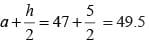Lower limit =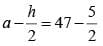= 44.5
[h = difference between any two marks = 52 – 47 = 5]

Math Olympiad Test: Statistics- 2 - Question 3

### Tallys are usually marked in a bunch of:

Detailed Solution for Math Olympiad Test: Statistics- 2 - Question 3

Tallies are usually marked in groups of five. The first four tallies are marked vertically and the fifth tally is marked diagonally across the first 4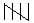.

Math Olympiad Test: Statistics- 2 - Question 4

The mid–value and upper limit of a class interval are 41 and 47 respectively. The class size will be:

Detailed Solution for Math Olympiad Test: Statistics- 2 - Question 4

Upper limit = mid value +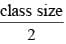47 = 41 +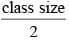∴ Class size = (47 – 41) × 2
= 12

Math Olympiad Test: Statistics- 2 - Question 5

The x-and y-axes in the histogram represent:

Detailed Solution for Math Olympiad Test: Statistics- 2 - Question 5

x-axis represents class interval, y-axis represents frequency.

Math Olympiad Test: Statistics- 2 - Question 6

In the ‘more–than’ type of ogive the cumulative frequency is plotted against:

Detailed Solution for Math Olympiad Test: Statistics- 2 - Question 6

More than distribution of cumulative frequency plot is a kind of Ogive frequency distribution in which the frequencies are decreasing from top to bottom.
So, we consider lower limits as abscissa and more than cumulative frequencies as ordinate.

Math Olympiad Test: Statistics- 2 - Question 7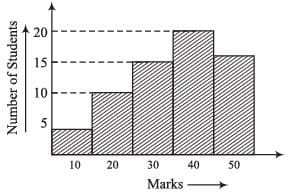The frequency of students is highest in the class interval:

Detailed Solution for Math Olympiad Test: Statistics- 2 - Question 7

∵ The class interval, 30 – 40, has highest peak,
∴ It has highest frequency.

Math Olympiad Test: Statistics- 2 - Question 8

The mean of x1, x2, ……., xn is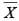, then the value of: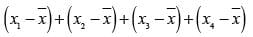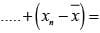Detailed Solution for Math Olympiad Test: Statistics- 2 - Question 8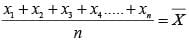⇒ x1 + x2 + x3 + x4 ……. + xn = n⇒ x1 + x2 + x3 …… + xn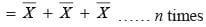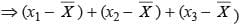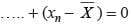Math Olympiad Test: Statistics- 2 - Question 9

If each number in (Prob - 17) is multiplied by k, the new mean will be:

Detailed Solution for Math Olympiad Test: Statistics- 2 - Question 9

Given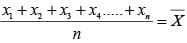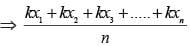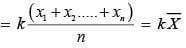Math Olympiad Test: Statistics- 2 - Question 10

The mean of 10 numbers is 16. If two consecutive numbers are excluded, the new mean is 18. The sum of the excluded numbers is:

Detailed Solution for Math Olympiad Test: Statistics- 2 - Question 10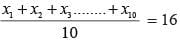⇒ x1 + x2 …… + x10 = 16 ……. (i)
Let x2 and x3 are removed, then,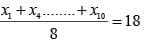⇒ x1 + x4 + ….. + x10 = 144 …….(ii)
Now, Eq. (i) – (ii)
x2 + x3 = 160 – 144 = 16

Math Olympiad Test: Statistics- 2 - Question 11

The sum of deviations of a set of n values x1, x2, …… xn measured from 50 is – 10 and the sum of deviations of the values from 46 is 70. The value of n is:

Detailed Solution for Math Olympiad Test: Statistics- 2 - Question 11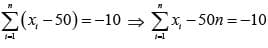…(i)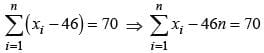…(ii)
Subtracting eq. (i), from eq. (ii),
46n – (–50 n) = 70 – (–10)
⇒ 4n = 80
⇒ n = 20

Math Olympiad Test: Statistics- 2 - Question 12

The mean of the following distribution is: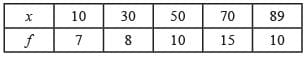Detailed Solution for Math Olympiad Test: Statistics- 2 - Question 12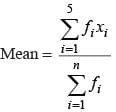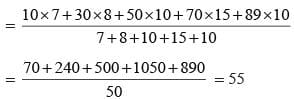Math Olympiad Test: Statistics- 2 - Question 13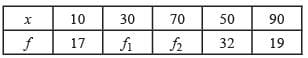Total = 120
Mean =  50
f1, f2 =

Detailed Solution for Math Olympiad Test: Statistics- 2 - Question 13

f1 + f2 + 17 + 32 + 19 = 120
⇒ f1 + f2 = 52 …(i)
Mean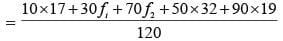⇒ 50 × 120 = 170 + 1600 + 1710 + + 30f1 + 70f2
⇒ 30f1 + 70f2 = 2520
⇒ 371 + 7f2 = 252 ……..(ii)
From eq (i) and eq (ii).
f1 = 28, f2 = 24

Math Olympiad Test: Statistics- 2 - Question 14

The new median, of the following data, if 37 is replaced by 5.
7, 9, 16, 25, 31, 36, 37, 39, 40, 42, 43

Detailed Solution for Math Olympiad Test: Statistics- 2 - Question 14

If 37 ⟶ 5, then, the new data will be:
5, 7, 9, 16, 25, 31, 36, 39, 40, 42, 43
∵ No. of numbers = 11
∴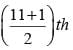= 6th number will be the median.

Math Olympiad Test: Statistics- 2 - Question 15

The mode of the following data is:
29, 40, 41, 46, 45, 44, 43, 29, 40, 41, 46, 44,  44, 47, 49, 53, 29, 57, 44, 43, 41, 28, 16, 26.

Detailed Solution for Math Olympiad Test: Statistics- 2 - Question 15

∵ 44 is repeated 4 times, i.e., maximum no. of times.
∴ Mode = 44

## Mathematics Olympiad for Class 9

1 videos|43 tests
 Use Code STAYHOME200 and get INR 200 additional OFF Use Coupon Code
Information about Math Olympiad Test: Statistics- 2 Page
In this test you can find the Exam questions for Math Olympiad Test: Statistics- 2 solved & explained in the simplest way possible. Besides giving Questions and answers for Math Olympiad Test: Statistics- 2, EduRev gives you an ample number of Online tests for practice

## Mathematics Olympiad for Class 9

1 videos|43 tests